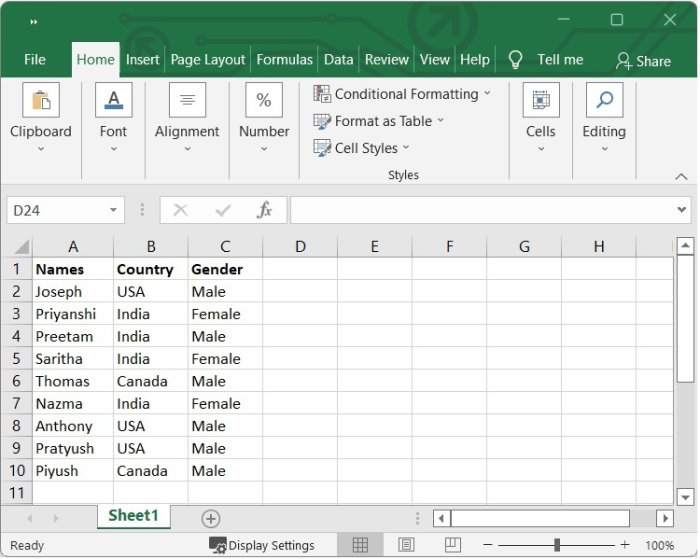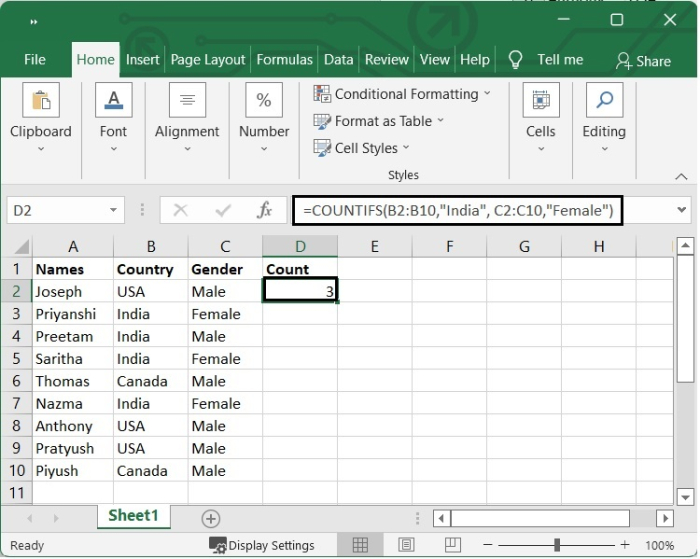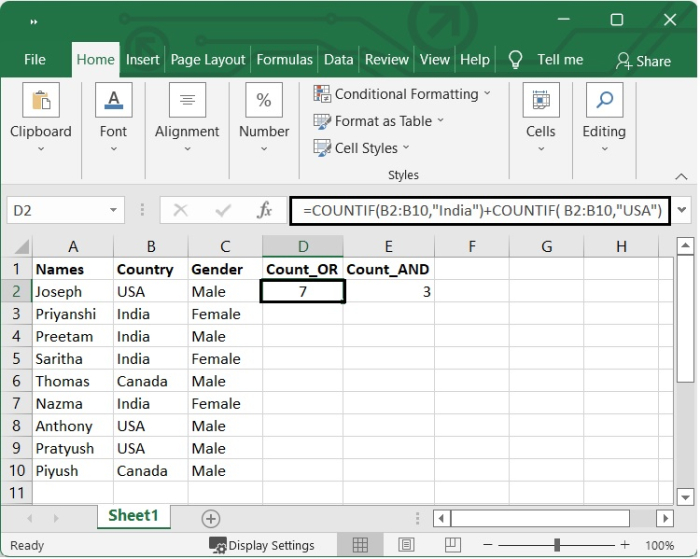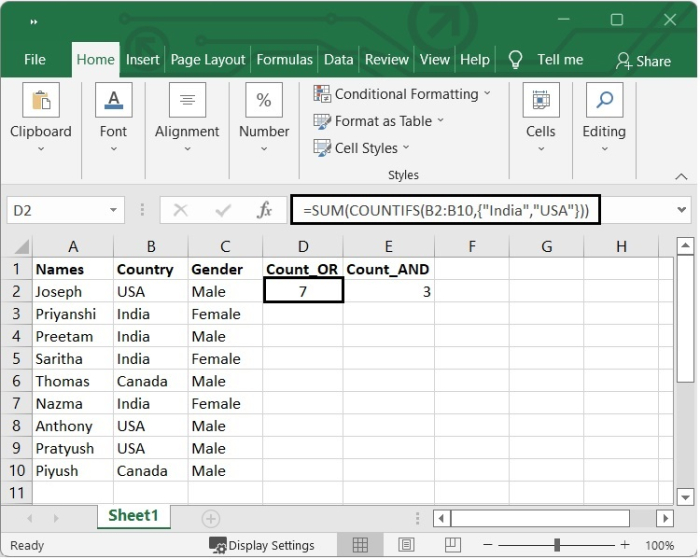# Excel COUNTIFS Function â€“ Count cells with multiple criteria â€“ AND logic and OR

In Excel, the COUNTIFS function allows you to count cells based on one or more criteria using AND logic. This can be done in the normal course of events. The COUNTIFS Function is one of the statistical functions that is found in Excel. COUNTIFS will count the number of cells that meet a single criterion or multiple criteria in the same or separate ranges. This can be done in either the same or a different range.

The primary distinction between COUNTIF and COUNTIFS is that the former is intended for counting cells that meet a single criterion within a given range, while the latter is flexible enough to evaluate several criteria within the same or other ranges.

In this tutorial, you are going to learn how to count cells with AND and OR logic.

## Count Cells using AND Logic

Since the COUNTIFS function in Excel is meant to count only those cells for which all of the stated conditions are TRUE, this is the scenario that presents the least amount of difficulty to deal with. Because the AND function in Excel operates in this manner, we refer to it as the AND logic.

Letâ€™s go through an example and understand how the count occurs using AND logic.

Step 1

In our example, we have some employees name with their country name and their gender. See the below given image.Step 2

We want to count the employees who are female and from India. So we will use the following formula.

=COUNTIFS(B2:B10,"India", C2:C10,"Female")


After adding the formula, press Enter and we will get the result as shown in the following image.## Count Cells using OR Logic

You might have ever been in a position where you needed to count more than one value from a single column or a range of cells. In that case, it involves using multiple conditions and OR logic when making your count. In this scenario, you have the option of adding up several COUNTIF formulas or using a SUM and COUNTIFS.

### Adding two or more COUNTIF

Letâ€™s suppose we want to count those employees who are either from USA or from India. We can add two COUNTIF as follows.

=COUNTIF(B2:B10,"India")+COUNTIF( B2:B10,"USA")


After pressing Enter, we will get the result as shown in the following image.### Using SUM and COUNTIFS Function

We can use the following formula to count the employees either from India and USA.

=SUM(COUNTIFS(B2:B10,{"India","USA"}))


After pressing Enter, we will get the result as shown in the following image.## Conclusion

In this tutorial, you learnt how to use the Excel COUNTIFS function to count cells using AND and OR logic.